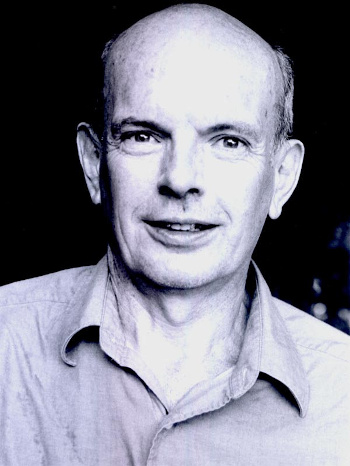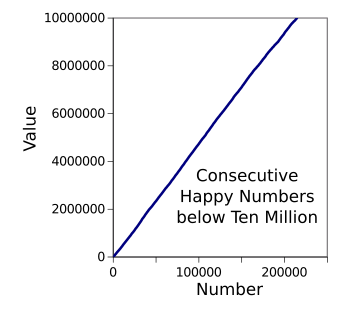### Happy Numbers

May 2, 2022

The first mathematics that children learn is the sequence of natural numbers, 1, 2, 3, et cetera. This sequence, also called the sequence of positive integers, is sequence A000027 of the On-Line Encyclopedia of Integer Sequences located at oeis.org. The On-Line Encyclopedia of Integer Sequences was created by mathematician, Neil Sloane (b. 1939), who started a card file of common integer sequences while a student in 1965. Sloane received his Ph.D. from Cornell University (Ithaca, New York) in 1967, and he published a book, "A Handbook of Integer Sequences," containing 2,400 sequences in 1973. The online version was started in 1996 with 10,000 sequences, now growing at about forty per day to reach more than 350,000 sequences at the time of this writing. The sequence with the lowest index number is A000001, the number of groups of order n.Neil Sloane (b. 1939).

The title of Sloane's doctoral dissertation was "Lengths of Cycle Times in Random Neural Networks." Sloane joined Bell Labs in 1968, becoming an AT&T Fellow in 1998, and retiring in 2012. Sloan is an IEEE Fellow, receiving the IEEE Richard W. Hamming Medal in 2005, a Fellow of the American Mathematical Society, and a member of the National Academy of Engineering.

Sloane shared some of his favorite integer sequences on the occasion of his 75th birthday in 2014.

Wikimedia Commons image by Laine Whitcomb.

Since there are so many cataloged sequences, it's difficult to find a novel sequence. However, this didn't deter my colleague, Steve Sund, from finding A181975, the numbers 1, 2, 3, 4, 5, 4, 3, 2, repeated, expressed as a(n) = abs(mod((n+3),8)-4)+1, a representation of a triangle waveform. I haven't contributed a sequence, but I've published blog articles about some interesting sequences.
• Partitioning, February 21, 2011
• Phyllotaxis, April 4, 2012
• Smith Numbers, May 9, 2012
• Holey Numbers, August 1, 2012
• Conway Numbers, October 10, 2012
• The Twin Prime Conjecture, June 3, 2013
• The Knight's Tour, March 7, 2014
• The Yellowstone Sequence, February 12, 2015
• Numerology, March 2, 2015
• Bus Stop Simulation, November 5, 2018
• Crazy Number Sequences, January 28, 2019
• Armstrong Numbers, November 2, 2020
• The Seven Sisters, March 22, 2021
• Sum of Three Cubes, March 14, 2022

The integer sequence of the number of days between these articles is 408, 35, 84, 70, 236, 277, 342, 18, 1344, 84, 644, 140, 357. Most of the integer sequences are named for what they represent, as this short list demonstrates.

• A005843, the non-negative even numbers.
• A005408, the non-negative odd numbers.
• A000040, the prime numbers.
• A000290, the squares.
• A077800, the twin primes.
• A000041, the partition numbers.

Others are named after their originator.

• A005188, the Armstrong numbers. These are the numbers that are the sum of their own digits each raised to the power of the number of digits.
• A033307, the digits of the Champernowne constant, which is simply the concatenation of all the numbers, written in order (i.e., 12345678910111213141516...
• A000215, the Fermat numbers. These are numbers of the form a(n) = 2^(2^n) + 1.
• A000045, Fibonacci numbers. This sequence starts with 0 and 1, and each subsequent term is the sum of the two preceding terms.
• A007318, Pascal's triangle (The binomial coefficients).
• A005597, from the first Hardy–Littlewood conjecture, the digits of the twin prime constant.
• A006753, a Smith number is a composite number for which the sum of its digits is equal to the sum of the digits in its prime factorization. You can read more about these and find source code for a generating program in an earlier article (Smith Numbers, May 9, 2012).

Others have imaginative names derived from their properties.

• A005188, the Armstrong numbers are also known as the narcissistic numbers.
• A006753, the Smith numbers are also called the joke numbers.
• A001744, the holey numbers. These are numbers whose written digits all have topological holes; e.g., 0, 4, 6. 8, and 9.
• A002113, the palindrome numbers, which are numbers the same when written forwards and backwards.
• A011541, the taxicab numbers. These numbers, first noticed by Srinivasa Ramanujan (1887-1920), are numbers that can be expressed as a sum of two positive integer cubes in n distinct ways. The name derives from the number 1729 seen on a taxicab by G.H. Hardy (1877-1947).
• A165134, knight tour numbers. These are the number of knight's paths visiting each square of an n-by-n chessboard exactly once.
• A098550, the Yellowstone sequence. This sequence has a graph that suggests the eruption of geysers in Yellowstone National Park in the U.S. state, Wyoming.

If you need a respite from the real mathematics of the world, you can vacation with A007770, the happy numbers. These are numbers that eventually give 1 when you go through the process of repeatedly replacing the number by the sum of the square of each digit. As an example, 31 is a happy number because 32 + 12 = 10 and 12 + 02 = 1. Further replacements will still yield 1. This concept can be generalized to any number base, there are happy primes (A035497), and the complementary unhappy numbers (A031177).One reason why your grandparents were so strange.

These are smiley balloon faces from a 1922 advertisement, but they seem more designed to induce nightmares than glee.

(Portions of an Archive.org image of a page from The Billboard, vol. 34, no. 11 (March 18, 1922), p. 20.)

Some integer sequences are interesting enough to encourage further research, and the happy numbers are one of these. While at Rutgers University (Piscataway, New Jersey), Justin Gilmer found that the lower density of this sequence is ≤ 0.1138 and the upper density is > 0.18577. Hao Pan has examined consecutive happy numbers, and Luca Onis has examined a variant of the happy numbers sequence given by A351327. The following table lists the fourteen consecutive happy number pairs less than a thousand,

 (31,32) (319,320) (637,638) (129,130) (367,368) (655,656) (192,193) (391,392) (912,913) (262,263) (565,566) (931,932) (301,302) (622,623)

I've modified a C language program from the Rosetta Code website to produce a file of happy numbers up to an intended limit (source code here). The program is quite efficient, checking ten million numbers on my not-so-fast desktop computer in 1.34 seconds. There are 1,418,854 happy numbers through ten million, consistent with the density range found by Gilmer. A companion program for finding the top member of consecutive happy number pairs can be found here, and the following graph shows their surprising constant density.graph of the top value of consecutive happy number pairs below ten million, as calculated by my C language program.

There are 214,600 such pairs.

(Created using Gnumeric.)### References:

1. Alex Bellos, "Neil Sloane: the man who loved only integer sequences," The Guardian, October 7, 2014.
2. N. J. A. Sloane,A Handbook of Integer Sequences, Academic Press (New York: 1973), xiii+206 pp., ISBN 0-12-648550-X. A PDF file of the introductory chapters can be found here.
3. Justin Gilmer, "On the density of happy numbers," arXiv, March 1, 2015.
4. Hao Pan, "Consecutive happy numbers," arXiv, July 7, 2006.
5. Luca Onnis, "On a variant of the happy numbers and their generalizations," arXiv, February 25, 2022.
6. C program for finding happy numbers at rosettacode.org.

Linked Keywords: Mathematics; child; children; learning; learn; integer sequence; natural number; positive integer; A000027; On-Line Encyclopedia of Integer Sequences; oeis.org; mathematician; Neil Sloane (b. 1939); index card; card file; student; Doctor of Philosophy; Ph.D.; Cornell University (Ithaca, New York); academic publishing; publish; book; Internet; online; index (publishing); A000001; group (mathematics); randomness; random; artificial neural network; Bell Labs; AT&T Corporation; Fellow<; retirement; retire; Institute of Electrical and Electronics Engineers; IEEE; Richard W. Hamming Medal; American Mathematical Society; National Academy of Engineering; birthday; Laine Whitcomb; colleague; Steve Sund; A181975; triangle waveform; blog; day; A005843; negative number; non-negative; parity (mathematics); even number; A005408; odd number; A000040; prime number; A000290; square number; A077800; twin prime; A000041; originator; A005188; Armstrong number; addition; sum; numerical digit; exponentiation; raise to a power; A033307; Champernowne constant; concatenation (mathematics); A000215; Fermat number; A000045; Fibonacci number; A007318; Pascal's triangle; binomial coefficient; A005597; first Hardy–Littlewood conjecture; twin prime constant; A006753; Smith number; composite number; prime factorization; source code; imagination; imaginative; A005188; narcissistic number; A006753; joke number; A001744; topology; topological; torus; hole; A002113; palindrome; A011541; taxicab number; Srinivasa Ramanujan (1887-1920); cube (algebra); taxicab; G.H. Hardy (1877-1947); A165134; knight's tour; knight's paths; square (geometry); chessboard; A098550; Cartesian coordinate system; graph; geyser; Yellowstone National Park; U.S. state; Wyoming; respite; vacation; A007770; happy number; radix; number base; A035497; A031177; grandparent; strange; smiley; balloon; face; advertising; advertisement; nightmare; pleasure; glee; Archive.org; research; number density; A351327; C language program; Rosetta Code; computer file; happy.c; desktop computer; happy consecutive.c; calculation; calculated; Gnumeric.